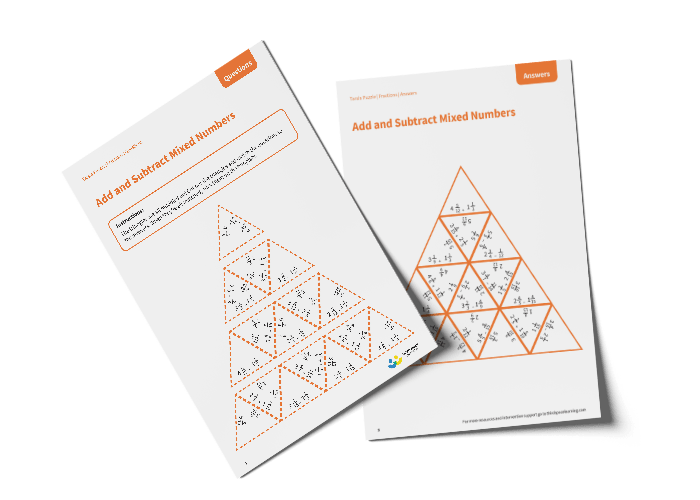# Tarsia Puzzle Add and Subtract Mixed Numbers (Year 5)

The triangles in the tarsia puzzle have all been mixed up. In this adding and subtracting mixed numbers tarsia puzzle, pupils will need to match the addition or subtraction question to the correct answer to create one large triangle.

This Year 5 add and subtract mixed numbers tarsia puzzle worksheet contains:

• One question sheet
• One template sheet

Year 5

Fractions